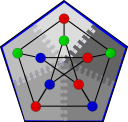#Boost C++ Libraries

...one of the most highly regarded and expertly designed C++ library projects in the world.

#Unique R-MAT generator

``` template<typename RandomGenerator, typename Graph>
class unique_rmat_iterator
{
public:
typedef std::input_iterator_tag iterator_category;
typedef std::pair<vertices_size_type, vertices_size_type> value_type;
typedef const value_type& reference;
typedef const value_type* pointer;
typedef void difference_type;

unique_rmat_iterator();
unique_rmat_iterator(RandomGenerator& gen, vertices_size_type n,
edges_size_type m, double a, double b, double c,
double d, bool permute_vertices = true);
// Iterator operations
reference operator*() const;
pointer operator->() const;
unique_rmat_iterator& operator++();
unique_rmat_iterator operator++(int);
bool operator==(const unique_rmat_iterator& other) const;
bool operator!=(const unique_rmat_iterator& other) const;
};
```

This class template implements a generator for R-MAT graphs [CZF04], suitable for initializing an adjacency_list or other graph structure with iterator-based initialization. An R-MAT graph has a scale-free distribution w.r.t. vertex degree and is implemented using Recursive-MATrix partitioning. The edge list produced by this iterator is guaranteed not to contain parallel edges.

# Where Defined

<boost/graph/rmat_graph_generator.hpp>

# Constructors

```unique_rmat_iterator();
```

Constructs a past-the-end iterator.

```unique_rmat_iterator(RandomGenerator& gen, vertices_size_type n,
edges_size_type m, double a, double b, double c,
double d, bool permute_vertices = true,
EdgePredicate ep = keep_all_edges());
```

Constructs an R-MAT generator iterator that creates a graph with n vertices and m edges. a, b, c, and d represent the probability that a generated edge is placed of each of the 4 quadrants of the partitioned adjacency matrix. Probabilities are drawn from the random number generator gen. Vertex indices are permuted to eliminate locality when permute_vertices is true. ep allows the user to specify which edges are retained, this is useful in the case where the user wishes to refrain from storing remote edges locally during generation to reduce memory consumption.

# Example

```#include <boost/graph/adjacency_list.hpp>
#include <boost/graph/rmat_graph_generator.hpp>
#include <boost/random/linear_congruential.hpp>

typedef boost::unique_rmat_iterator<boost::minstd_rand, Graph> RMATGen;

int main()
{
boost::minstd_rand gen;
// Create graph with 100 nodes and 400 edges
Graph g(RMATGen(gen, 100, 400, 0.57, 0.19, 0.19, 0.05,),
RMATGen(), 100);
return 0;
}
```

# Bibliography

 [CZF04] D Chakrabarti, Y Zhan, and C Faloutsos. R-MAT: A Recursive Model for Graph Mining. In Proceedings of 4th International Conference on Data Mining, pages 442--446, 2004.

Copyright (C) 2009 The Trustees of Indiana University.

Authors: Nick Edmonds and Andrew Lumsdaine